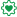# Qlik Design Blog

All about product and Qlik solutions: scripting, data modeling, visual design, extensions, best practices, etc.

Announcements
Coming your way, the Qlik Data Revolution Virtual Summit. October 27-29. REGISTEREmployee

## Getting all data cells from app.createCube()

As I was working on one of my latest mashups, I had a task, to get all cells with numerous sets of dimensions and measures, with a HyperCube out of Qlik Sense. The worst part is that one of my sets, had a total of 3+ million cells!

We know that the initial HyperCube returns only up to ~10k cells, So the question is how do we paginate to get the rest or even get them all in one call!

We also know that, most of the Capability API methods are using promises, something very helpful, especially if we make a lot of calls to Qlik Sense and expect to return the results only when all of the promises have been resolved. This is where promises “.all()” come into play.

Even though ES6 has promises build in, not all browsers support it yet, so I will use Angular's \$q to handle those promises.

First we have to make the initial call and get the maximum rows and columns. We start by creating the cube with the dimensions

```app.createCube({
qDimensions : qDimensions,
qMeasures : qMeasures
}).then(function(model) {

```

Once we have the model ready, we make the initial call to get the first set of results and get the HyperCube info like total columns and total rows

```model.getHyperCubeData('/qHyperCubeDef', [{qTop: 0, qWidth: 20, qLeft: 0, qHeight: 500}]).then(function(data) {

```

Once we have that we can determine how many total pages do we have

```var columns = model.layout.qHyperCube.qSize.qcx;
var totalheight = model.layout.qHyperCube.qSize.qcy;
var pageheight = Math.floor(10000 / columns);
var numberOfPages = Math.ceil(totalheight / pageheight);

```

Now, if we got all of the results, we should end the method and return those results

```if (numberOfPages==1) {
deferred.resolve(data.qDataPages.qMatrix);
}

```

Otherwise, we should get the data from all pages and then return the results! Promises in full glory!!!

```var Promise = \$q;
var promises = Array.apply(null, Array(numberOfPages)).map(function(data, index) {
var page = {
qTop: (pageheight * index) + index,
qLeft: 0,
qWidth: columns,
qHeight: pageheight,
index: index
};
return model.getHyperCubeData('/qHyperCubeDef', [page]);
}, this);
Promise.all(promises).then(function(data) {
for (var j=0; j<data.length; j++) {
for (var k=0; k<data.qDataPages.qMatrix.length; k++) {
qTotalData.push(data.qDataPages.qMatrix)
}
}
deferred.resolve(qTotalData);
});

```

This is how we get all of the results for a given set of Dimensions and Measures.

If you want to paginate just create a function that passes the page+1*500 to the qInitialDataFetch like

```app.createCube({
qDimensions : qDimensions,
qMeasures : qMeasures,
qInitialDataFetch : [{
qTop : (pageIndex)*500,
qLeft : 0,
qHeight : 500,
qWidth : 20
}]

```

Or

```model.getHyperCubeData('/qHyperCubeDef', [{qTop: (pageIndex)*500, qWidth: 20, qLeft: 0, qHeight: 500 }]).then(function(data) {

```
Tags (2)